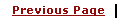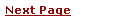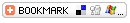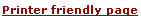C - Structured DatatypesLearning C
C Function References
C Useful Resources# C - Structured Datatypes• A structure in C is a collection of items of different types. You can think of a structure as a "record" is in Pascal or a class in Java without methods.

• Structures, or structs, are very useful in creating data structures larger and more complex than the ones we have discussed so far.

• Simply you can group various built-in data types into a structure.

• Object conepts was derived from Structure concept. You can achieve few object oriented goals using C structure but it is very complex.

Following is the example how to define a structure.

 ```struct student { char firstName; char lastName; char SSN; float gpa; }; ```

Now you have a new datatype called student and you can use this datatype define your variables of student type:

 ```struct student student_a, student_b; or an array of students as struct student students; ```

Another way to declare the same thing is:

 ```struct { char firstName; char lastName; char SSN; float gpa; } student_a, student_b; ```

All the variables inside an structure will be accessed using these values as student_a.firstName will give value of firstName variable. Similarly we can aqccess other variables.

# Structure Example:

Try out following example to understand the concept:

 ```#include struct student { char firstName; char lastName; char SSN; float gpa; }; main() { struct student student_a; strcpy(student_a.firstName, "Deo"); strcpy(student_a.lastName, "Dum"); strcpy(student_a.SSN, "2333234" ); student_a.gpa = 2009.20; printf( "First Name: %s\n", student_a.firstName ); printf( "Last Name: %s\n", student_a.lastName ); printf( "SNN : %s\n", student_a.SSN ); printf( "GPA : %f\n", student_a.gpa ); } ```

This will produce following results:

 ```First Name: Deo Last Name: Dum SSN : 2333234 GPA : 2009.20 ```

# Pointers to Structs:

Sometimes it is useful to assign pointers to structures (this will be evident in the next section with self-referential structures). Declaring pointers to structures is basically the same as declaring a normal pointer:

 ```struct student *student_a; ```

To dereference, you can use the infix operator: ->.

 ```printf("%s\n", student_a->SSN); ```

# typedef Keyword

There is an easier way to define structs or you could "alias" types you create. For example:

 ```typedef struct{ char firstName; char lastName; char SSN; float gpa; }student; ```

Now you can use student directly to define variables of student type without using struct keyword. Following is the example:

 ```student student_a; ```

You can use typedef for non-structs:

 ```typedef long int *pint32; pint32 x, y, z; ```

x, y and z are all pointers to long ints

# Unions Datatype

Unions are declared in the same fashion as structs, but have a fundamental difference. Only one item within the union can be used at any time, because the memory allocated for each item inside the union is in a shared memory location.

Here is how we define a Union

 ```union Shape { int circle; int triangle; int ovel; }; ```

We use union in such case where only one condition will be applied and only one variable will be used.

# Conclusion:

• You can create arrays of structs.
• Structs can be copied or assigned.
• The & operator may be used with structs to show addresses.
• Structs can be passed into functions. Structs can also be returned from functions.
• Structs cannot be compared!
• Structures can store non-homogenous data types into a single collection, much like an array does for common data (except it isn't accessed in the same manner).
• Pointers to structs have a special infix operator: -> for dereferencing the pointer.